Ex 8.1

Serial order wise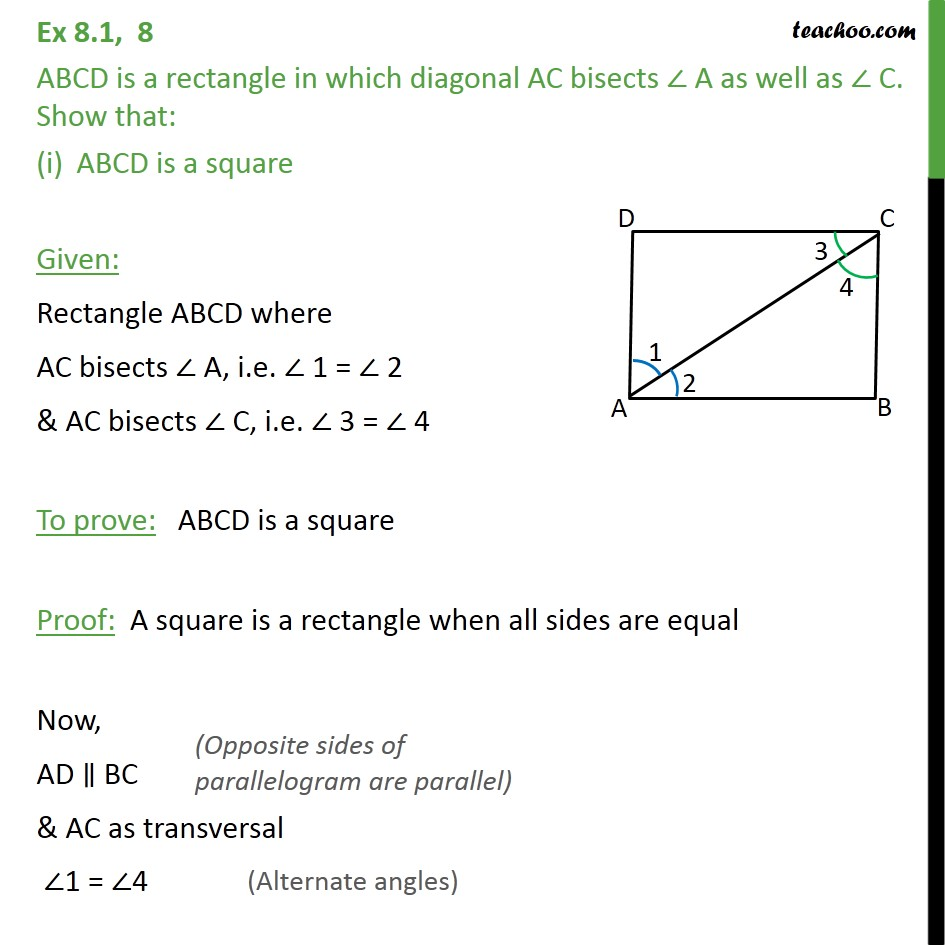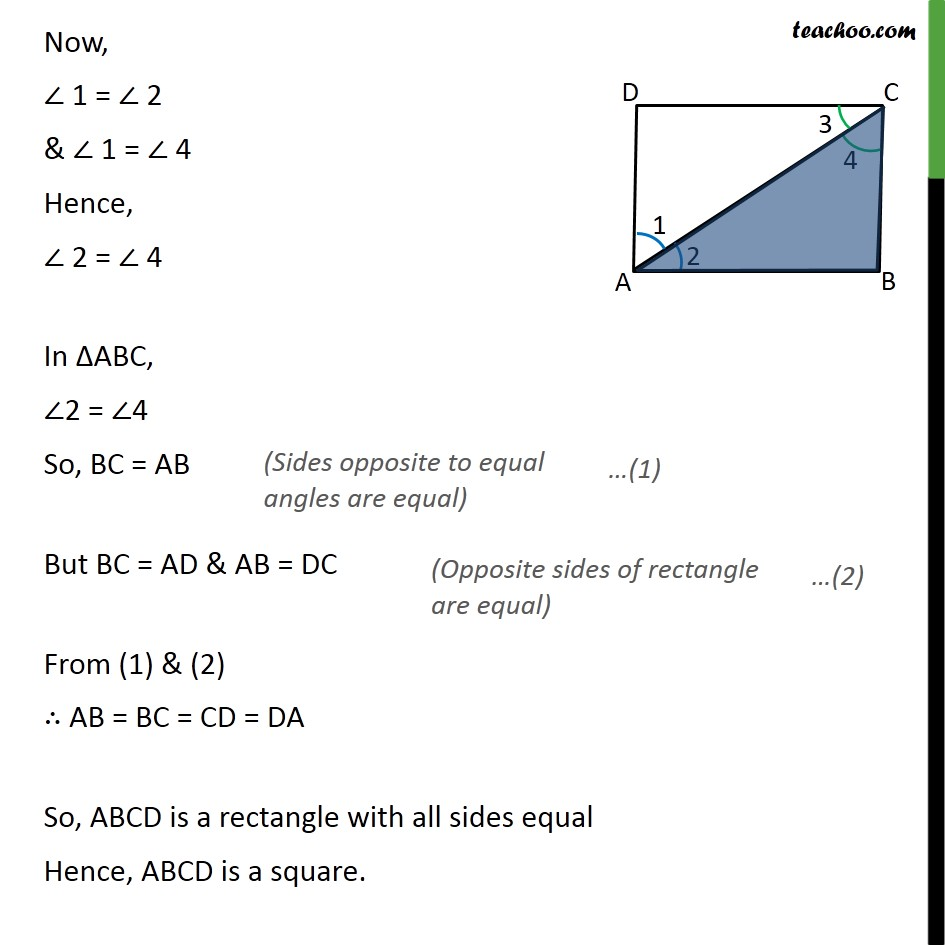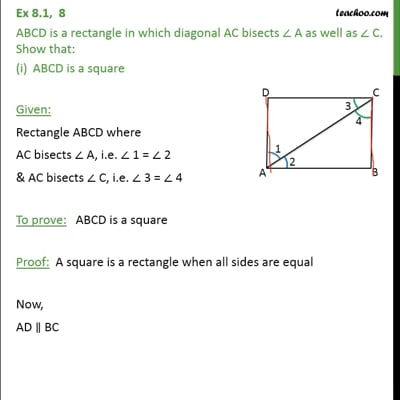This video is only available for Teachoo black users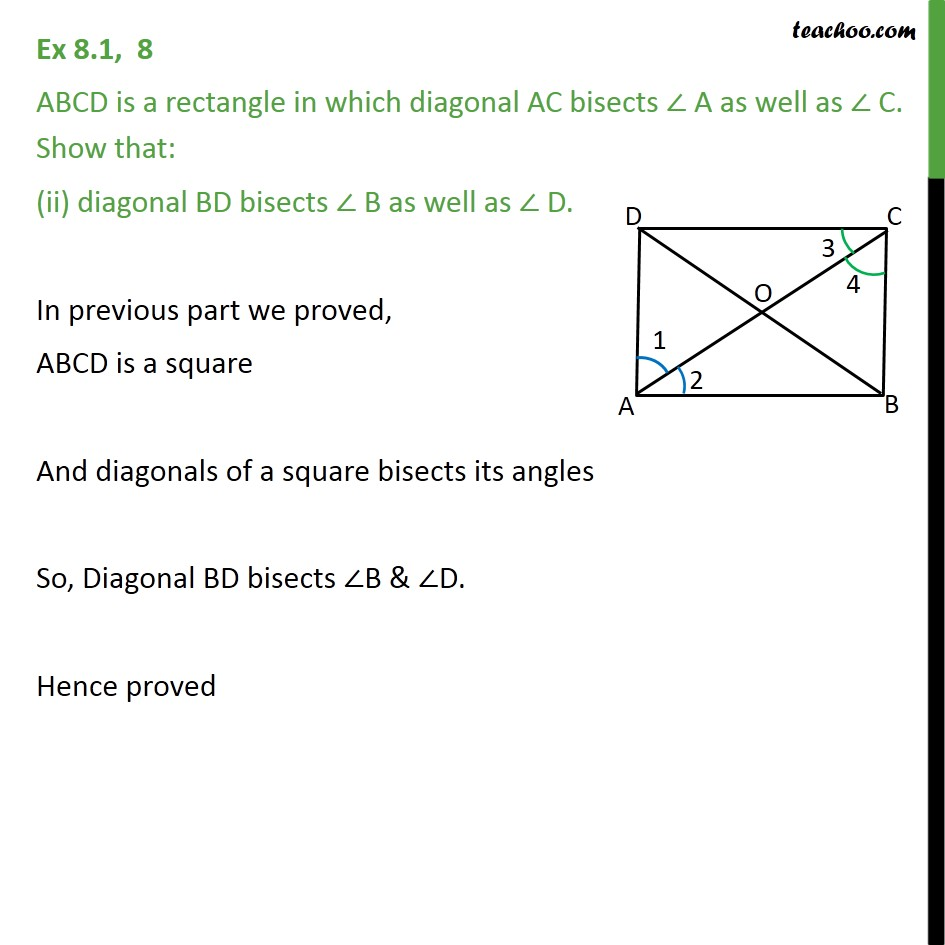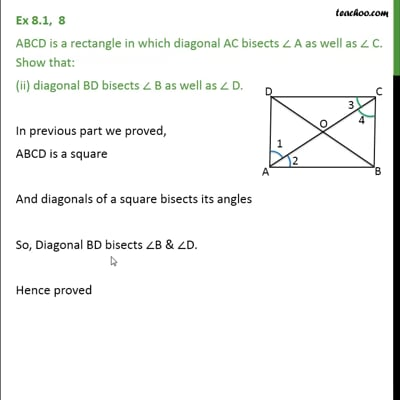This video is only available for Teachoo black users

Maths Crash Course - Live lectures + all videos + Real time Doubt solving!

### Transcript

Ex 8.1, 8 ABCD is a rectangle in which diagonal AC bisects A as well as C. Show that: ABCD is a square Given: Rectangle ABCD where AC bisects A, i.e. 1 = 2 & AC bisects C, i.e. 3 = 4 To prove: ABCD is a square Proof: A square is a rectangle when all sides are equal Now, AD BC & AC as transversal 1 = 4 Now, 1 = 2 & 1 = 4 Hence, 2 = 4 In ABC, 2 = 4 So, BC = AB But BC = AD & AB = DC From (1) & (2) AB = BC = CD = DA So, ABCD is a rectangle with all sides equal Hence, ABCD is a square. Ex 8.1, 8 ABCD is a rectangle in which diagonal AC bisects A as well as C. Show that: (ii) diagonal BD bisects B as well as D. In previous part we proved, ABCD is a square And diagonals of a square bisects its angles So, Diagonal BD bisects B & D. Hence proved